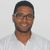# New to Qlik Sense

If you’re new to Qlik Sense, start with this Discussion Board and get up-to-speed quickly.

cancel
Showing results for
Did you mean:Contributor III

## Calculating condition Qlik Sense

Dear community,

I need help understanding how the DATA HANDLING - CALCULATION CONDITION of a chart works in Qlik Sense.

I have a table with a dimension calculated as follow:

=if((A) <= B,'OK','ATTENTION') as "A <= B"

and I need to write a condition that displays the table only when "A <= B" = 'ATTENTION' and if the condition is not satisfied  want to display a message. This condition ("A <= B" = 'ATTENTION') is not working, can someone please help me with this? Thanks

Labels (9)

• ### tables if columns

1 Solution

Accepted SolutionsContributor III
Author

I found the solution to my problem!!

The correct answer is: sum(if([A<=B]='ATTENTION',1,0) ) > 0

Thanks for the help

8 RepliesMVP

@g23  can you share a sample and the expected output ?

Regards,
Taoufiq ZARRA

"Please LIKE posts and "Accept as Solution" if the provided solution is helpful "

(you can mark up to 3 "solutions") 😉Partner - Contributor III

The calculation Condition is used to determine whether or not an object (e.g. chart) is to be displayed.

If you are trying to display only records with the value "ATTENTION", set analysis could help you.

Link to a post, where a similar question was solved:

Hope this helps,

TomášContributor III
Author

Here an example of data and the dimension calculated.

Hope this helps, thanksContributor III
Author

I tried applying this condition:

if([A<=B]='ATTENTION',1,0) but it's not workingMVP

@g23   How would you like to satisfy the condition? When user selects    'ATTENTION' from  [A<=B]?Partner - Contributor III

Instead of using calculation condition (which provides 2 options: display / not display all table values based on the result of the calculation), I would suggest to try defining the value of e.g. expression A in this way: if(A<=B,A,null()) .

Then if you turn off showing null values for this dimension (Uncheck Include null values), you will see only the values, that satisfy your condition.

The other option is to use set analysis in defining your dimensions / expressions.Contributor III
Author

I created a drop down input variable in which the user selects the dimension to display on tableContributor III
Author

I found the solution to my problem!!

The correct answer is: sum(if([A<=B]='ATTENTION',1,0) ) > 0

Thanks for the helpTags
Community Browser